Courses

# Test: Coordinate Geometry- 5

## 15 Questions MCQ Test General Aptitude for GATE 2020 | Test: Coordinate Geometry- 5

Description
This mock test of Test: Coordinate Geometry- 5 for GATE helps you for every GATE entrance exam. This contains 15 Multiple Choice Questions for GATE Test: Coordinate Geometry- 5 (mcq) to study with solutions a complete question bank. The solved questions answers in this Test: Coordinate Geometry- 5 quiz give you a good mix of easy questions and tough questions. GATE students definitely take this Test: Coordinate Geometry- 5 exercise for a better result in the exam. You can find other Test: Coordinate Geometry- 5 extra questions, long questions & short questions for GATE on EduRev as well by searching above.
QUESTION: 1

### If the point (x, y) is equidistant from the points (5, 1) and ( – 1, 5), then the relation between ‘x’ and ‘y’ is given by

Solution:

Explanation:

Let the point C(x,y)is equidistant from the points A (5, 1) and B(−1,5).(−1,5).

i.e. AC = BC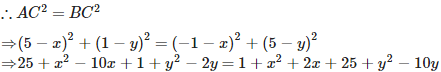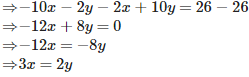QUESTION: 2

### The points A(4, – 1), B(6, 0), C(7, 2) and D(5, 1) are the vertices of a

Solution: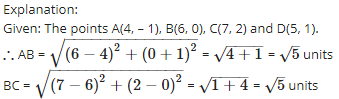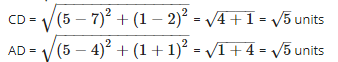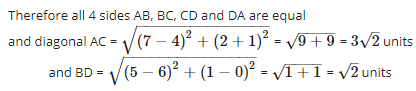Therefore diagonals AC and BD are not equal
Since, all sides are equal and both diagonals are not equal.
Therefore, the given quadrilateral is a rhombus.

QUESTION: 3

### The co – ordinates of the mid – point of the line joining the points (3p, 4) and ( – 2, 4) are (5, p). The value of ‘p’ is

Solution:

Explanation:

Let the coordinates of midpoint O(5,p) is equidistance from the points A(3p,4) and B(−2,4).( because O is the mid-point of AB)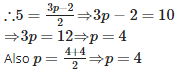QUESTION: 4

If the points (2, 3), (4, k) and (6, – 3) are collinear, then the value of ‘k’ is

Solution:

Explanation:

Let the points A (2, 3), B(4,k) and C(6,−3) be collinear.
If the points are collinear then area of triangle ABC formed by these three points is 0.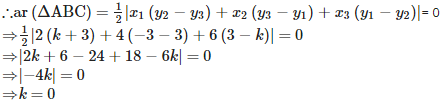QUESTION: 5

The distance of a point from the y – axis is called

Solution:

Explanation:

The distance of a point from the y – axis is the x (horizontal)  coordinate of the point and is  called abscissa.

QUESTION: 6

If the point P(2, 4) lies on a circle, whose centre is C(5, 8), then the radius of the circle is

Solution:

Explanation:The point P(2 , 4) is on the circle and C(5, 8) is its centre

Hence PC will be Radius of circle.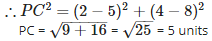QUESTION: 7

The perimeter of a triangle with vertices (0, 4), (0, 0) and (3, 0) is

Solution:

Explanation:

Given: the vertices of a triangle ABC, A(0, 4), B (0, 0) and C (3, 0).
∴ Perimeter of triangle ABC = AB + BC + AC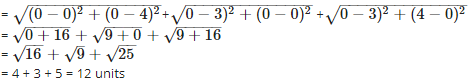QUESTION: 8

The co – ordinates of the point which divides the join of ( – 6, 10) and (3, – 8) in the ratio 2 : 7 is

Solution: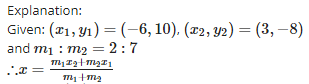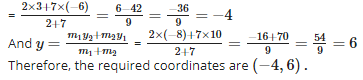QUESTION: 9

If the points (x, y), (1, 2) and (7, 0) are collinear, then the relation between ‘x’ and ‘y’ is given by

Solution: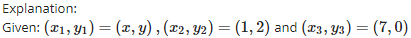and these are collinear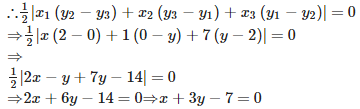QUESTION: 10

The distance of a point from the x – axis is called

Solution:

Explanation:The distance of a point from the x – axis is the y (vertical) coordinate of the point and is called ordinate.

QUESTION: 11

A circle has its centre at the origin and a point P(5, 0) lies on it. Then the point Q(8, 6) lies ________ the circle.

Solution: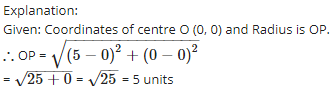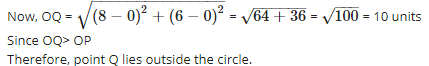QUESTION: 12

(0, 3), (4, 0) and ( – 4, 0) are the vertices of

Solution: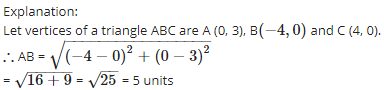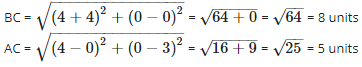Since, two sides are equal, therefore, ABC is an isosceles triangle.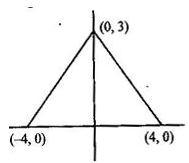QUESTION: 13

The ratio in which the point (1, 3) divides the line segment joining the points ( – 1, 7) and (4, – 3) is

Solution: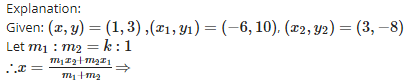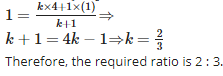QUESTION: 14

If the vertices of a triangle are (1, 1), ( – 2, 7) and (3, – 3), then its area is

Solution:

Explanation:

Given: (x1,y1)=(1,1),(x2,y2)=(−2,7) and (x3,y3)=(3,−3)=(3,−3), then the Area of trianlge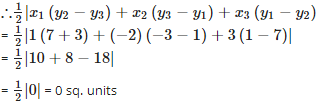Also therefore the three given points(vertices) are collinear

QUESTION: 15

The point of intersection of the x – axis and y – axis is called

Solution:

Explanation:The point of intersection of the x – axis and y – axis is called origin.The coordinates of origin are (0, 0).